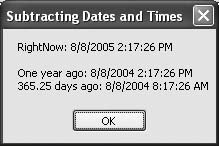# Recipe 7.13. Subtracting from Dates and Times

#### Problem

You want to subtract some amount of time from a date or time.

#### Solution

Sample code folder: Chapter 07\SubtractTime

Use the various "Add" functions of the Date object, passing negative values to subtract amounts of time.

#### Discussion

The Date object does not provide any "Subtract" functions for subtracting specific units of time. You can instead simply "add" negative amounts of time. The following code demonstrates how this works:

` Dim results As New System.Text.StringBuilder Dim rightNow As Date = Now results.AppendLine("RightNow: " & rightNow.ToString) results.AppendLine() results.AppendLine("One year ago: " & _    rightNow.AddYears(-1).ToString) results.AppendLine("365.25 days ago: " & _    rightNow.AddDays(-365.25).ToString) MsgBox(results.ToString()) `

Figure 7-13 shows the results of these negative time additions as displayed by the message box in the last line.

##### Figure 7-13. To subtract years, days, or other amounts of time, add negative quantitiesEach Date object does provide a Subtract() function, as discussed in Recipe 7.14. However, this function subtracts either another Date value or a TimeSpan. It is possible to create a TimeSpan given an amount of time and its units, but simply adding specific negative units of time is a very straightforward way to get the task accomplished.

Recipe 7.12 lists the various "Add" date methods.Visual Basic 2005 Cookbook: Solutions for VB 2005 Programmers (Cookbooks (OReilly))
ISBN: 0596101775
EAN: 2147483647
Year: 2006
Pages: 400

Similar book on Amazon